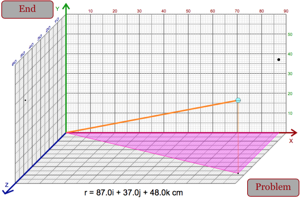Information About The Program
Length and Angle of 3d Vector (Homework)A program that will randomly generate a three dimensional vector. Students will be told the x, y, and z components of this vector and they must find the length of the vector and the angles the vector makes with the x-z plane and the x-axis.
Below are any Resources that go with this program